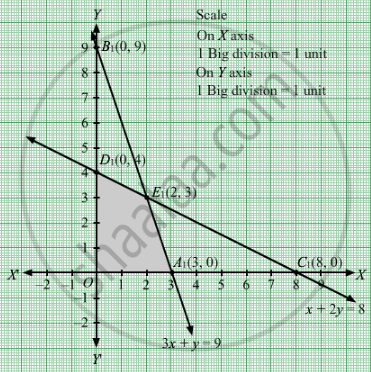# A Company Produces Two Types of Goods, a and B, that Require Gold and Silver.Each Unit of Type a Requires 3 Gm of Silver and 1 Gm of Gold While that of Type B Requires 1 Gm of Silver and 2 Gm of Gold. - Mathematics

Sum

A company produces two types of goods, A and B, that require gold and silver. Each unit of type A requires 3 gm of silver and 1 gm of gold while that of type B requires 1 gm of silver and 2 gm of gold. The company can produce 9 gm of silver and 8 gm of gold. If each unit of type A brings a profit of Rs 40 and that of type B Rs 50, find the number of units of each type that the company should produce to maximize the profit. What is the maximum profit?

#### Solution

Let goods of type A and y goods of type B were produced.
Number of goods cannot be negative.
Therefore, $x, y \geq 0$

The given information can be tabulated as follows:

 Silver(gm) Gold white(gm) Type A 3 1 Type B 1 2 Availability 9 8

Therefore, the constraints are

$3x + y \leq 9$
$x + 2y \leq 8$

If each unit of type A brings a profit of Rs 40 and that of type B Rs 50.Then, x goods of type A and y goods of type B brings a profit of Rs 40x and Rs 50y.
Total profit = Z = $40x + 50y$ which is to be maximised.
Thus, the mathematical formulat​ion of the given linear programmimg problem is
Max Z =   $40x + 50y$

subject to

$3x + y \leq 9$
$x + 2y \leq 8$

$x, y \geq 0$

First we will convert inequations into equations as follows :
3x + y = 9, x + 2y = 8, x = 0 and y = 0

Region represented by 3x + y ≤ 9:
The line 3x + y = 9 meets the coordinate axes at A1(3, 0) and B1(0, 9) respectively. By joining these points we obtain the line

3x + y = 9.  Clearly (0,0) satisfies the 3x + y = 9. So,the region which contains the origin represents the solution set of the inequation 3x + y ≤ 9.

Region represented by x + 2y ≤ 8:
The line x + 2y = 8 meets the coordinate axes at C1(8, 0) and D1(0, 4) respectively. By joining these points we obtain the line x + 2y = 8.   Clearly (0,0) satisfies the inequation x + 2y ≤ 8. So,the region which contains the origin represents the solution set of the inequation x + 2y ≤ 8.

Region represented by x ≥ 0 and y ≥ 0:
Since, every point in the first quadrant satisfies these inequations. So, the first quadrant is the region represented by the inequations x ≥ 0, and ≥ 0.
The feasible region determined by the system of constraints 3x + y ≤ 9, x + 2y ≤ 8, x ≥ 0, and y ≥ 0 are as follows.The corner points are O(0, 0), D1(0, 4), E1(2, 3), A1(3, 0)
The values of Z at these corner points are as follows

 Corner point Z = 40x + 50y O 0 D1 200 E1 230 A1 120

The maximum value of Z is 230 which is attained at E1(2, 3).
Thus, the maximum profit  is of Rs 230 obtained when 2 units of type A and 3 units of type B produced.

Concept: Graphical Method of Solving Linear Programming Problems
Is there an error in this question or solution?

#### APPEARS IN

RD Sharma Class 12 Maths
Chapter 30 Linear programming
Exercise 30.4 | Q 9 | Page 51